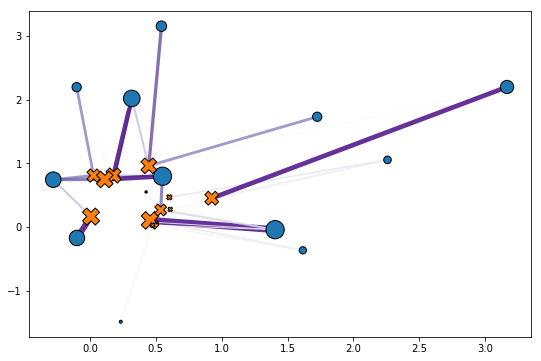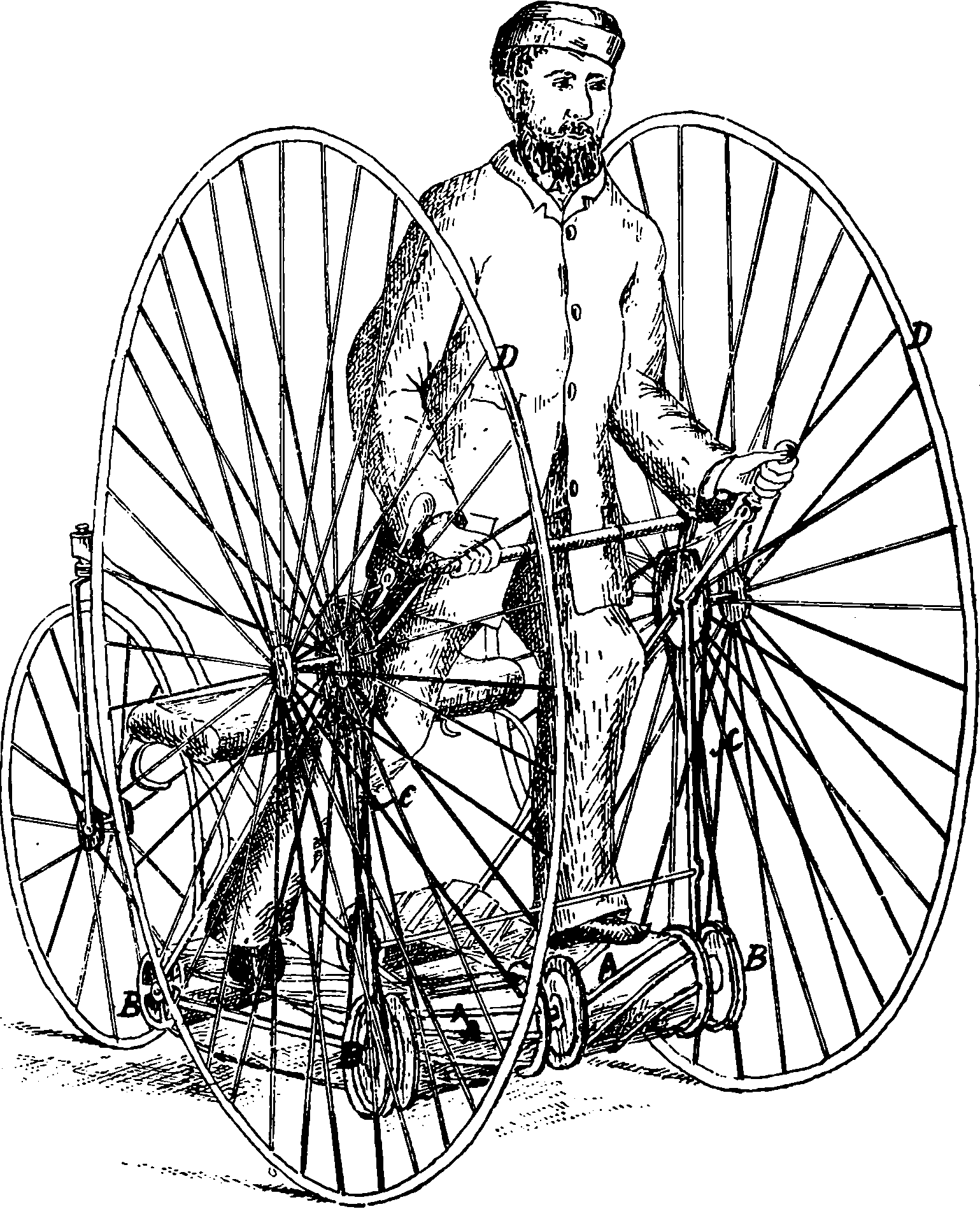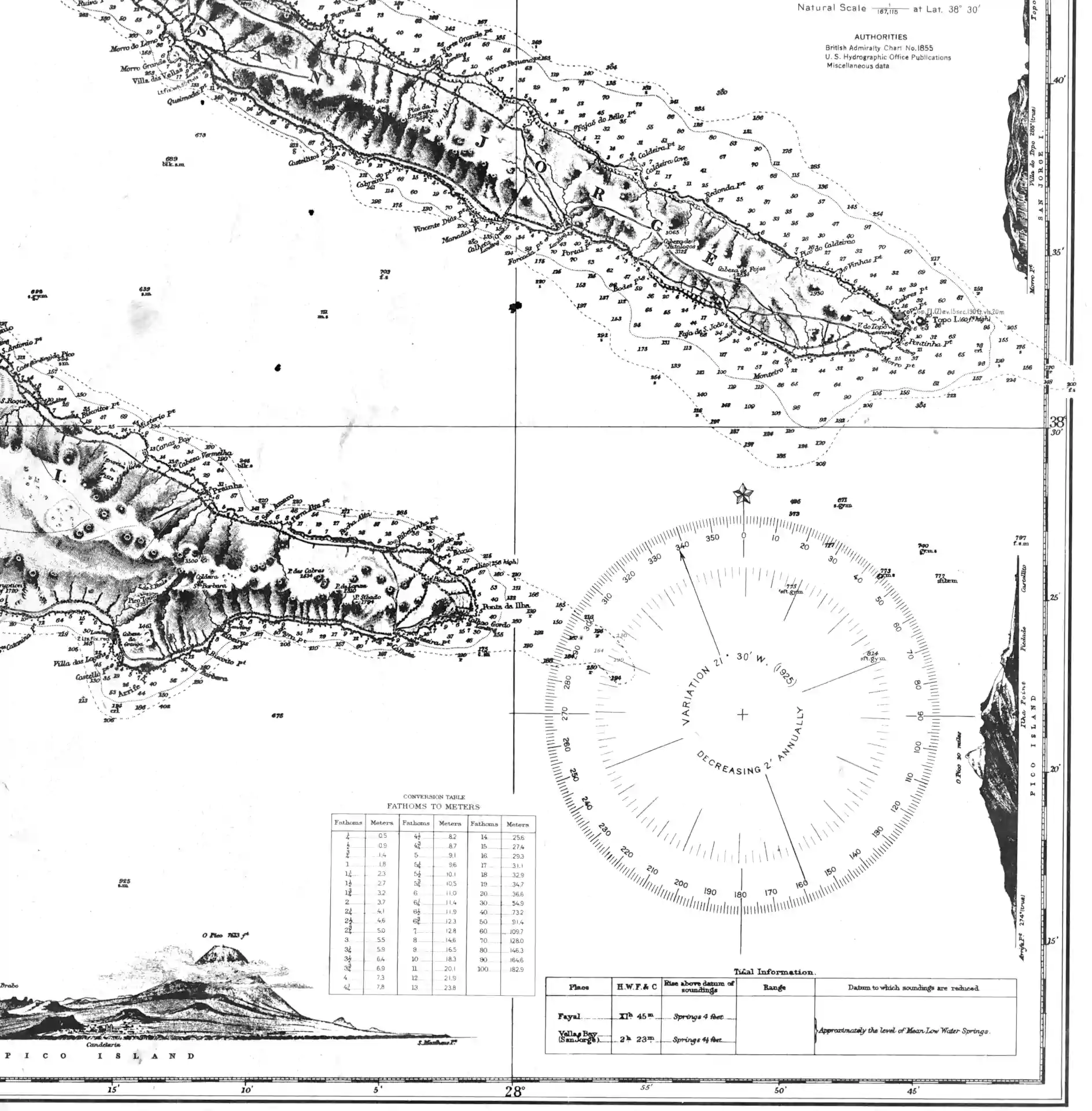# Optimal transport inference

## I feel the earth mover under my feet, I feel the ψ tumbling down, I feel my heart start to trembling, whenever you’re around (my barycentre)Doing inference where the probability metric measuring discrepancy between some target distribution and the implied inferential distribution is an optimal-transport one. Frequently intractable, but neat when we can get it. Sometimes we might get there by estimating the (gradients of) an actual OT loss, or even the transport maps implying that loss

Wasserstein GANs and OT Gans are argued to do an approximate optimal transport inference, indirectly.

See e.g. for a particular Bayes posterior approximation using OT.

## Minibatched

Daniel Daza in Approximating Wasserstein distances with PyTorch touches upon Fatras et al. (2020):

Optimal transport distances are powerful tools to compare probability distributions and have found many applications in machine learning. Yet their algorithmic complexity prevents their direct use on large scale datasets. To overcome this challenge, practitioners compute these distances on minibatches i.e., they average the outcome of several smaller optimal transport problems. We propose in this paper an analysis of this practice, which effects are not well understood so far. We notably argue that it is equivalent to an implicit regularization of the original problem, with appealing properties such as unbiased estimators, gradients and a concentration bound around the expectation, but also with defects such as loss of distance property.

## Tools## Linearized embedding

I learned about it from Bai et al. (2023) via Cheng-Soon Ong

Comparing K (probability) measures requires the pairwise calculation of transport-based distances, which, despite the significant recent computational speed-ups, remains to be relatively expensive. To address this problem, W. Wang et al. (2013) proposed the Linear Optimal Transport (LOT) framework, which linearizes the 2-Wasserstein distance utilizing its weak Riemannian structure. In short, the probability measures are embedded into the tangent space at a fixed reference measure (e.g., the measures’ Wasserstein barycenter) through a logarithmic map. The Euclidean distances between the embedded measures then approximate the 2-Wasserstein distance between the probability measures. The LOT framework is computationally attractive as it only requires the computation of one optimal transport problem per input measure, reducing the otherwise quadratic cost to linear. Moreover, the framework provides theoretical guarantees on convexifying certain sets of probability measures […], which is critical in supervised and unsupervised learning from sets of probability measures.

### OTT

Optimal Transport Tools (OTT) , a toolbox for all things Wasserstein (documentation):

The goal of OTT is to provide sturdy, versatile and efficient optimal transport solvers, taking advantage of JAX features, such as JIT, auto-vectorization and implicit differentiation.

A typical OT problem has two ingredients: a pair of weight vectors a and b (one for each measure), with a ground cost matrix that is either directly given, or derived as the pairwise evaluation of a cost function on pairs of points taken from two measures. The main design choice in OTT comes from encapsulating the cost in a Geometry object, and [bundling] it with a few useful operations (notably kernel applications). The most common geometry is that of two clouds of vectors compared with the squared Euclidean distance, as illustrated in the example below:

import jax
import jax.numpy as jnp
from ott.tools import transport
# Samples two point clouds and their weights.
rngs = jax.random.split(jax.random.PRNGKey(0),4)
n, m, d = 12, 14, 2
x = jax.random.normal(rngs, (n,d)) + 1
y = jax.random.uniform(rngs, (m,d))
a = jax.random.uniform(rngs, (n,))
b = jax.random.uniform(rngs, (m,))
a, b = a / jnp.sum(a), b / jnp.sum(b)
# Computes the couplings via Sinkhorn algorithm.
ot = transport.solve(x, y, a=a, b=b)
P = ot.matrix

The call to sinkhorn above works out the optimal transport solution by storing its output. The transport matrix can be instantiated using those optimal solutions and the Geometry again. That transport matrix links each point from the first point cloud to one or more points from the second, as illustrated below.

To be more precise, the sinkhorn algorithm operates on the Geometry, taking into account weights a and b, to solve the OT problem, produce a named tuple that contains two optimal dual potentials f and g (vectors of the same size as a and b), the objective reg_ot_cost and a log of the errors of the algorithm as it converges, and a converged flag.obtained coupling from Cuturi et al. (2022)

### POT

This open source Python library provide several solvers for optimization problems related to Optimal Transport for signal, image processing and machine learning.

Website and documentation: https://PythonOT.github.io/

Source Code (MIT): https://github.com/PythonOT/POT

POT provides the following generic OT solvers (links to examples):

POT provides the following Machine Learning related solvers:

Some other examples are available in the documentation.

### GeomLoss

The GeomLoss library provides efficient GPU implementations for:

GeomLoss functions are available through the custom PyTorch layers SamplesLoss, ImagesLoss and VolumesLoss which allow you to work with weighted point clouds (of any dimension), density maps and volumetric segmentation masks.